# 漫画：以后有面试官问你快速排序，你就干脆把我这篇熬夜写的文章扔给他！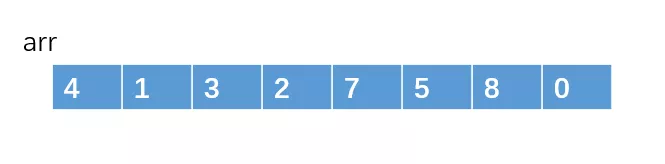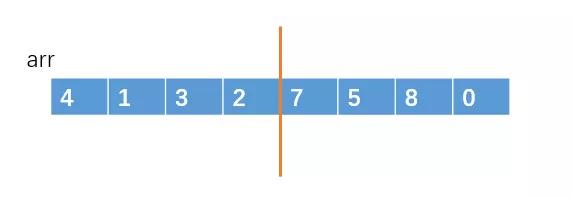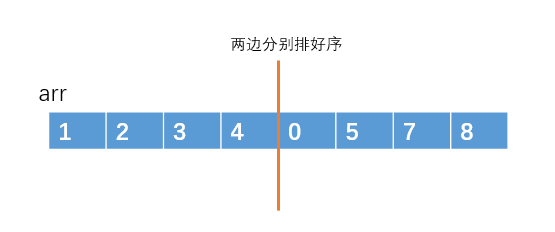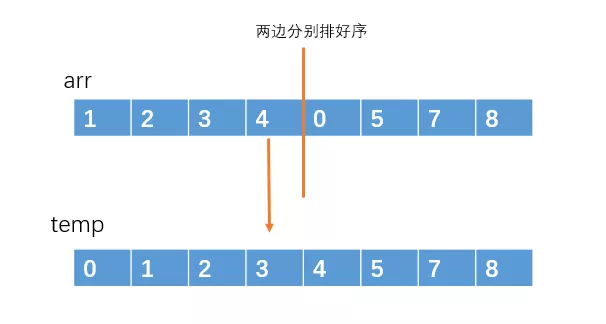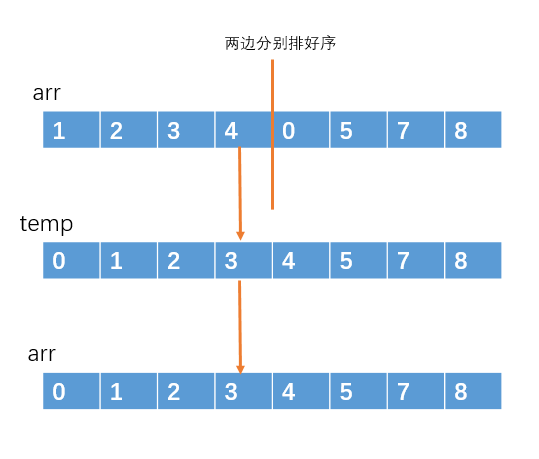### 快速排序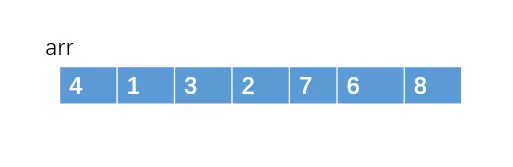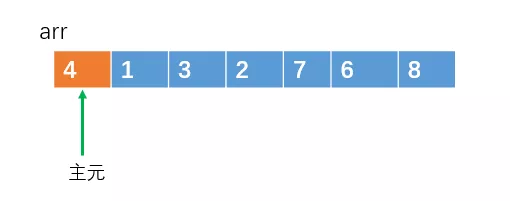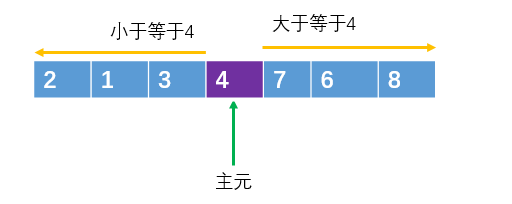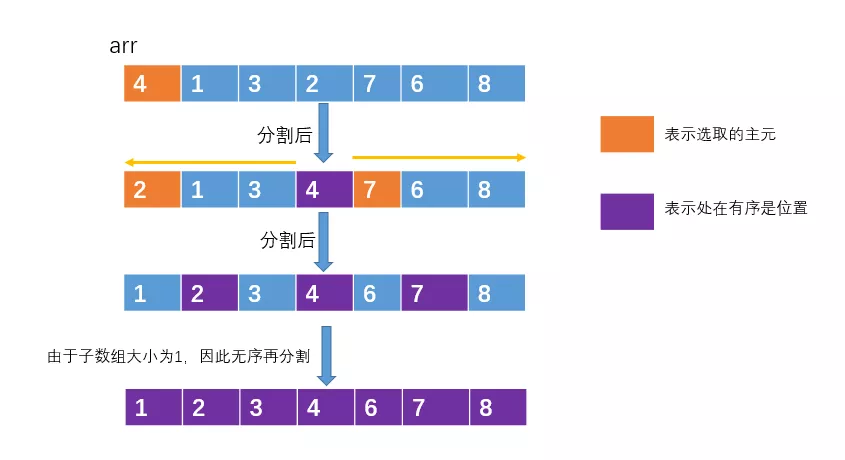### 分割操作：单向调整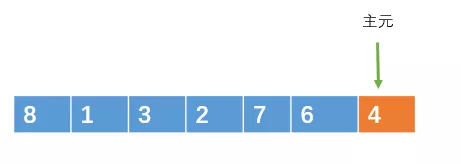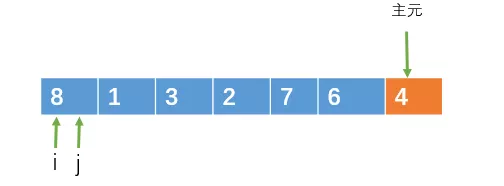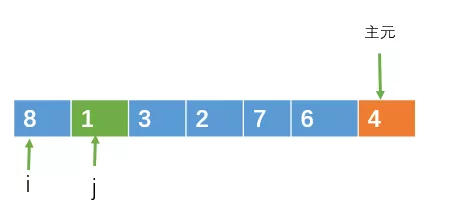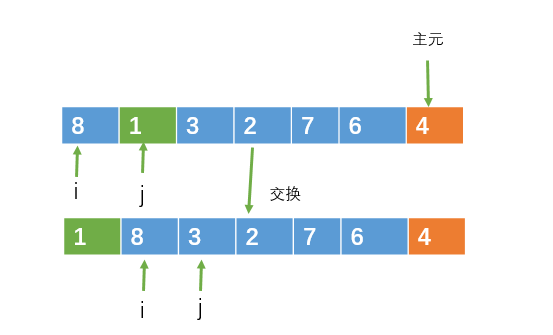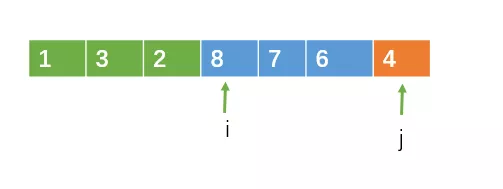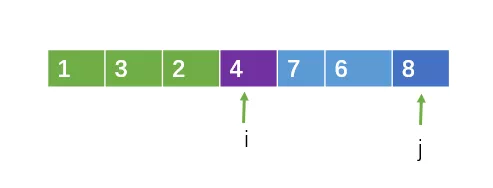//分割操作：方法一，单向调整
int partion(int a[], int left, int right)
{
int temp,pivot;//pivot存放主元
int i,j;
i = left;
pivot = a[right];
for(j = left;j < right;j++)
{
if(a[j] < pivot)
{  //交换值
temp = a[i];
a[i] = a[j];
a[j] = temp;
i++;
}
}
a[right] = a[i];
a[i] = pivot;
return i;//把主元的下标返回
}
//快速排序
void QuickSort(int a[], int left, int right)
{
int center;
int i,j;
int temp;
if(left < right)
{
center = partion(a,left,right);
QuickSort(a,left,center-1);//左半部分
QuickSort(a,center+1,right);//右半部分
}
}


### 分割操作：双向调整8被重复交换了很多次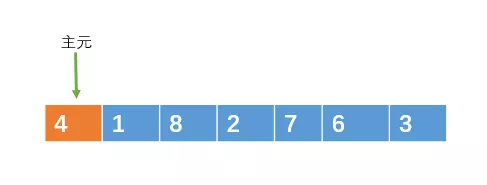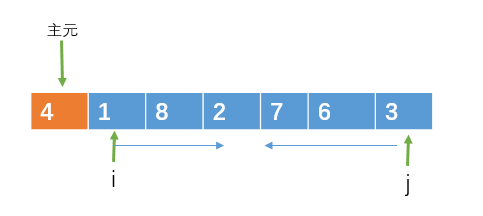i 向右遍历的过程中，如果遇到大于或等于主元的元素时，则停止移动，j向左遍历的过程中，如果遇到小于或等于主元的元素则停止移动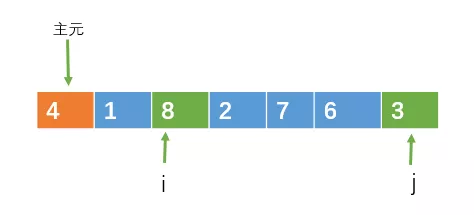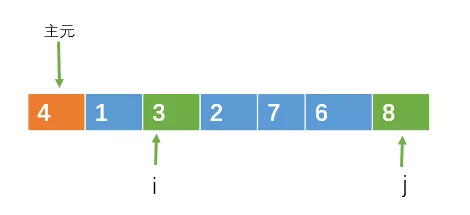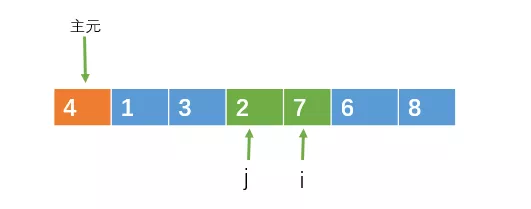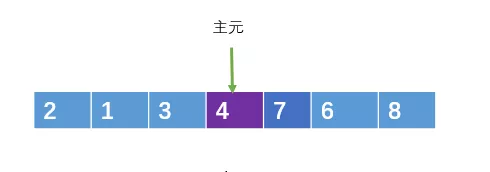方法二：双向扫描
int partition2( int[] arr, int left, int right)
{
int pivot = arr[left];
int i = left + 1;
int j = right;
while(true)
{
//向右遍历扫描
while(i <= j && arr[i] <= pivot) i++;
//向左遍历扫描
while(i <= j && arr[j] => pivot) j--;
if(i >= j)
break;
//交换
int temp = arr[i];
arr[i] = arr[j];
arr[j] = temp;
}
//把arr[j]和主元交换
arr[left] = arr[j];
arr[j] = povit;
return j;
}


### 时间复杂度### 稳定性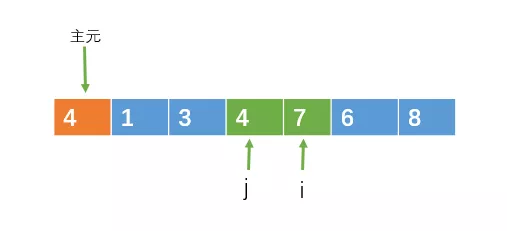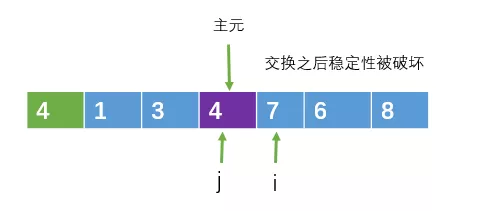//随机选取主元
int random_partition(int[] arr, int left, int right)
{
i = random(left, right);//随机选取一个位置
//在把这个位置的元素与ar[left]交换
swap(arr[i], arr[left]);

return partition(arr, left, right);
}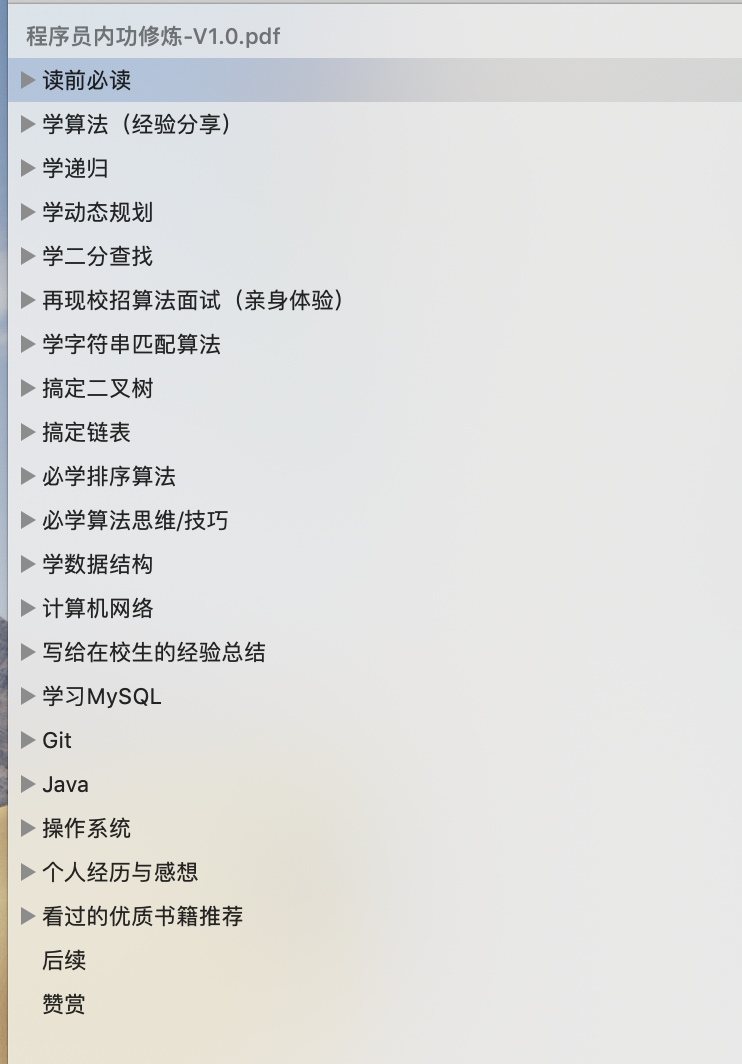### 兄dei，如果觉得我写的不错，不妨帮个忙

1、关注我的原创微信公众号「帅地玩编程」，每天准时推送干货技术文章，专注于写算法 + 计算机基础知识（计算机网络+ 操作系统+数据库+Linux），听说关注了的不优秀也会变得优秀哦。

2、给俺点个赞呗，可以让更多的人看到这篇文章，顺便激励下我，嘻嘻。

### 作者简洁

©️2019 CSDN 皮肤主题: 程序猿惹谁了 设计师: 上身试试